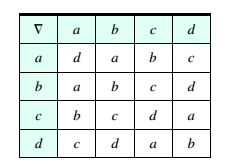Chapter 8.3, Problem 57ESMathematical Excursions (MindTap C...

4th Edition
Richard N. Aufmann + 3 others
ISBN: 9781305965584

Solutions

Chapter
SectionMathematical Excursions (MindTap C...

4th Edition
Richard N. Aufmann + 3 others
ISBN: 9781305965584
Textbook Problem

Consider the set of elements { a , b , c , d } with the operation V as given in the table below.For example, to compute c ∇ d , find c in the left column and align it with d in the top row. The result is a . Is the group commutative?

To determine

Consider the set of elements {a,b,c,d} with the operation as given in the following table

 ∇ a b c d a d a b c b a b c d c b c d a d c d a b

In order to compute cd , find c in the left column and align it with d in the top row. The result is a .

Is the group commutative?

Explanation

Given: The set of elements {a,b,c,d} with the operation as given in the following table

 ∇ a b c d a d a b c b a b c d c b c d a d c d a b

Calculation:

We have the set of elements {a,b,c,d

Still sussing out bartleby?

Check out a sample textbook solution.

See a sample solution

The Solution to Your Study Problems

Bartleby provides explanations to thousands of textbook problems written by our experts, many with advanced degrees!

Get Started

In the problem 1-4, solve the equations 3x14x9=57

Mathematical Applications for the Management, Life, and Social Sciences

In Exercises 722, evaluate the expression. 21. 161/4 81/3

Applied Calculus for the Managerial, Life, and Social Sciences: A Brief Approach

Solve the equations in Exercises 126. 2x3x2x3x+1=0

Finite Mathematics and Applied Calculus (MindTap Course List)

In Exercises 15-28, construct a truth table for the compound proposition. p(qr)

Finite Mathematics for the Managerial, Life, and Social Sciences